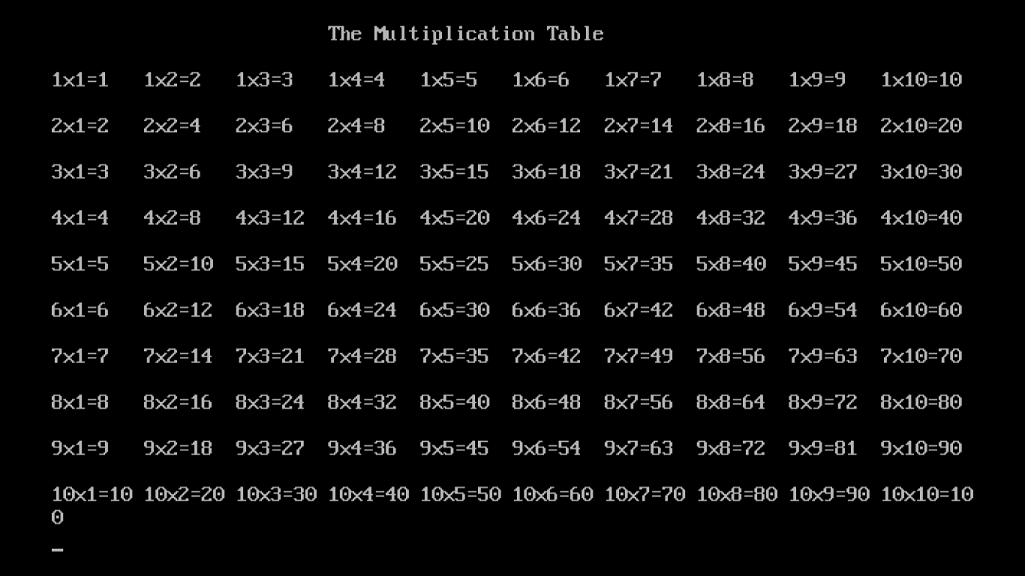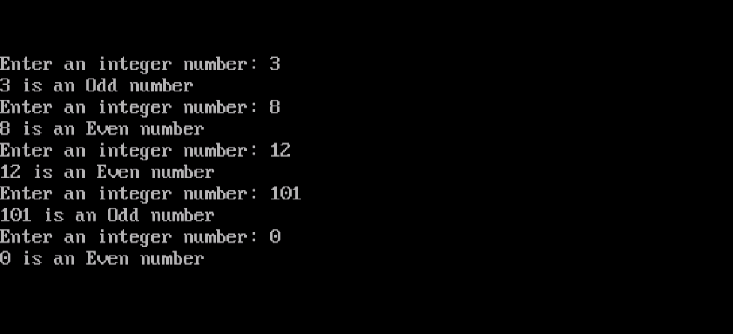Home System Programming Learn about For Loop and While Loop in C++ Programming

# Learn about For Loop and While Loop in C++ Programming

0
3824Now, an important topic that we (as programmers) can’t live without: Loops. So, bear with us, and read it, and you won’t regret! Promise! What are Loops? A Loop is a tool that executes a portion of code repeatedly either for a specific number of times or while a condition is true.

for Loop
The for loop repeatedly executes a portion of code (usually referred to as the Loop Body) a certain number of times.

Syntax
The syntax of the for loop is as follows:

```for (INITIALIZATION; CONDITION; UPDATE)
{
LOOP  BODY
}
```

Where
the INITIALIZATION section is an expression that initializes the loop variable.
CONDITION is a test expression that checks whether to execute the loop body one more time or not, and
the UPDATE section is where the loop variable is updated before testing for a new iteration.

Note
As in if statements: if the for loop body consists of only one statement, we can omit the curly braces (but we can still use them). In case of multiple-statements loop body, using the curly braces is a must.

Example
Your younger brother, who has a math exam tomorrow, asked you to review the multiplication table with him. You need to print the multiplication table for numbers from 1 to 10. How would achieve this to get an output screen like the one below?!Okay, consider the following code:

```#include<iostream.h>
#include<conio.h>
void main()
{
clrscr();
cout << endl << "\t\t\t The Multiplication Table\n\n";
for (int i=1;i<=10;i++)
{
for(int j=1;j<=10;j++)
cout << i << "x" << j << "=" << i*j << "\t";
cout << endl;
}
getch();
}
```

Actually, we used two for loops here, one from each type: single-statement body, and multiple-statements body.

• The outer loop for(int i=1;i<=10;i++)uses a loop variable i that is first initialized to 1
1. The condition is checked: i<=10 evaluates to true, so the loop body is executed for the first time.
2. After executing the loop body, the update section increments the loop variable.
3. Again, the condition is checked. i (which is now equal to 2) is less than or equal to 10, so another iteration will be executed.
4. The steps b and c will be repeated until i becomes 11. At this point, the condition will be false, and the loop exits.
• The inner for loop uses another variable j that also iterates over the range from 1 to 10. In each iteration, this loop performs a multiplication operation and prints its results.
• The outer loop represents the rows (lines). Notice that the first operand remains the same within a line of output. This is the loop variable of the outer loop.
• The inner loop manages the horizontal movement within the line. Notice that each field (column) will have the same value of the second operand in all lines. This is the loop variable of the inner loop.

Pretty easy, huh?while Loop
The while Loop uses a different logic. It continues to execute its body as long as a specific condition is met. The condition could be any expression that evaluates to either true or false (mind you, if the condition you use will always evaluate to true, the loop won’t exit, and the program will go into infinite loop.

Syntax

```while (CONDITION)
{
LOOP  BODY
}
```

Again, as in the for loop, if the while loop body consists of a single statement, we can omit the curly braces.

Example
Back to the Odd-Even program. What we are going to do here in this modified version is to repeatedly ask the user to enter integer numbers, this should continue until the user enters 0. Once the program sees 0 as input, it will exit.

Consider the following code:

```#include<iostream.h>
#include<conio.h>
void main()
{
int x=1;
clrscr();
while (x!=0)
{
cout << "Enter an integer number: ";
cin >> x;
if (x % 2 == 0)
cout<<x<<" is an Even number\n";
else
cout<<x<<" is an Odd number\n";
}
}
```

Let’s execute the program, and see what we will get:Here, the number of iterations the loop will execute is totally left to the user’s choice; it may be two, five, eleven, or just one. Note that we have initialized x to 1 to prevent the loop from exiting when testing the condition for the first time. So, to sum up, when the number of iteration is unknown in advance, the while loop will be a good choice.

while when acting like for
Being suitable for cases wherein the number of iteration is unknown in advance doesn’t mean it can’t work if the number is known. In other words, the while loop can also do the job of the for loop. The following example will show you that.

Example
Let’s re-write the Multiplication Table program using the while loop.

```#include<iostream.h>
#include<conio.h>
void main()
{
int j,i=1;
clrscr();
cout << endl << "\t\t\t The Multiplication Table\n\n";
while (i<=10)
{
j=1;
while(j<=10)
{
cout << i << "x" << j << "=" << i*j << "\t";
j++;
}
cout << endl;
i++;
}
getch();
}
```

As you can see, for the while loop to act as a for loop, we need to use the same three sections in the for loop definition: initialization, condition, and update. First the variable i is defined and initialized to 1, then the condition is tested, and in the last statement of the loop body, the variable i gets incremented. Exactly as we did in the for loop, but the for loop offered a more compact form that squeezed the three statements in one line.

Summary